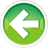# 4.10 — Introduction to if statements

Consider a case where you’re going to go to the market, and your roommate tells you, “if they have strawberries on sale, buy some”. This is a conditional statement, meaning that you’ll execute some action (“buy some”) only if the condition (“they have strawberries on sale”) is true.

Such conditions are common in programming, as they allow us to implement conditional behavior into our programs. The simplest kind of of conditional statement in C++ is called an if statement. An if statement allows us to execute one (or more) lines of code only if some condition is true.

The simplest if statement takes the following form:

```if (condition) statement;
```

For readability, this is more often written as following:

```if (condition)
statement;
```

A condition (also called a conditional expression) is an expression that evaluates to a Boolean value.

If the condition of an if statement evaluates to Boolean value true, then the subsequent statement is executed. If the condition instead evaluates to Boolean value false, the subsequent statement is skipped.

A sample program using an if statement

Given the following program:

Here’s output from one run of this program:

```Enter an integer: 0
The value is zero
```

Let’s examine how this works in more detail.

First, the user enters an integer. Then the condition x == 0 is evaluated. The equality operator (==) is used to test whether two values are equal. Operator== returns true if the operands are equal, and false if they are not. Since x has value 0, and 0 == 0 is true, this expression evaluates to true.

Because the condition has evaluated to true, the subsequent statement executes, printing You entered zero.

Here’s another run of this program:

```Enter an integer: 5
```

In this case, x == 0 evaluates to false. The subsequent statement is skipped, the program ends, and nothing else is printed.

Warning

If statements only conditionally execute a single statement.

Executing multiple statements conditionally

To execute multiple statements conditionally, place the statements between curly braces.

Running this program:

```Enter an integer: 4
You entered: 4
The value is positive
```
```Enter an integer: 0
```

We talk about what these curly braces are in lesson 6.1 -- Compound statements (blocks).

If-else

Given the above example, what if we wanted to tell the user that the number they entered was non-zero?

We could write something like this:

Or this:

Both of these programs are more complex than they need to be. Instead, we can use an alternative form of the if statement called if-else. If-else takes the following form:

```if (condition)
true_statement;
else
false_statement;
```

If the condition evaluates to Boolean true, true_statement executes. Otherwise false_statement executes.

Let’s amend our previous program to use an if-else.

Now our program will produce the following output:

```Enter an integer: 0
The value is zero
```
```Enter an integer: 5
The value is non-zero
```

Chaining if statements

Sometimes we want to check if several things are true or false in sequence. We can do so by chaining an if statement to a prior if-else, like so:

The less than operator (<) is used to test whether one value is less than another. Similarly, the greater than operator (>) is used to test whether one value is greater than another. These operators both return Boolean values.

Here’s output from a few runs of this program:

```Enter an integer: 4
The value is positive
```
```Enter an integer: -3
The value is negative
```
```Enter an integer: 0
The value is zero
```

Note that you can chain if statements as many times as you have conditions you want to evaluate. We’ll see an example in the quiz where this is useful.

Boolean return values and if statements

In the previous lesson (4.9 -- Boolean values), we wrote this program using a function that returns a Boolean value:

Let’s improve this program using an if statement:

Two runs of this program:

```Enter an integer: 5
Enter another integer: 5
5 and 5 are equal
```
```Enter an integer: 6
Enter another integer: 4
6 and 4 are not equal
```

In this case, our conditional expression is simply a function call to function isEqual, which returns a Boolean value.

Non-Boolean conditionals

In all of the examples above, our conditionals have been either Boolean values (true or false), Boolean variables, or functions that return a Boolean value. What happens if your conditional is an expression that does not evaluate to a Boolean value?

In such a case, the conditional expression is converted to a Boolean value: non-zero values get converted to Boolean true, and zero-values get converted to Boolean false.

Therefore, if we do something like this:

This will print “hi”, since 4 is a non-zero value that gets converted to Boolean true, causing the statement attached to the if to execute.

We’ll continue our exploration of if statements in future lesson 7.2 -- If statements and blocks.

Quiz time

Question #1

A prime number is a whole number greater than 1 that can only be divided evenly by 1 and itself. Write a program that asks the user to enter a single digit integer. If the user enters a single digit that is prime (2, 3, 5, or 7), print “The digit is prime”. Otherwise, print “The digit is not prime”.

Show Hint

Show Solution4.11 -- Compound statements and nested blocksIndex4.9 -- Boolean values